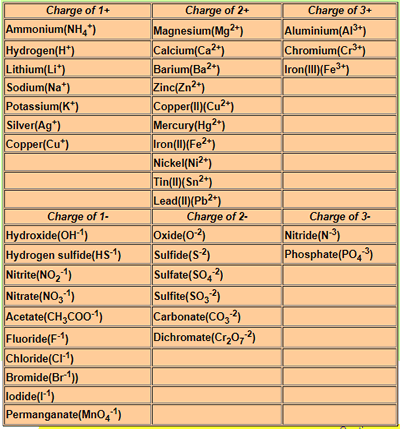Overall equations for precipitation reactions. Writing the overall equation for a precipitation reaction involves skills in writing ionic formulae and balancing chemical equations. Lets start with an example. A solution of lead nitrate is mixed with a solution of potassium iodide.to produce solid lead iodide and soluble potassium nitrate. Step 1) Write the equation with words placing the reactants on the left and the products on the right of the arrow. lead nitrate + potassium iodide => lead iodide + potassium nitrate Stpe 2) Replace the name with the chemical formula of each reactant and product in the equation. Pb(NO3)2 + KI => PbI2 + KNO3 Step 3) Balance the equation Pb(NO3)2 + 2KI => PbI2 + 2KNO3 Step 4) Give the states of all reactants and products. Keep in mind that the precipitate will be a solid and will have the symbol (s) while all the other species are in solution and will have the symbol (aq). Pb(NO3)2(aq) + 2KI (aq)=> PbI2 (s) + 2KNO3 (aq) 1) Solutions of copper nitrate and sodium carbonate react to produce solid copper carbonate and soluble sodium nitrate. Solution 2) Solutions of aluminium nitrate and sodium hydroxide react to produce solid aluminium hydroxide and soluble sodium nitrate. Solution 3) Solutions of copper sulfate and lead nitrate react to produce solid lead sulfate and soluble copper nitrate. 4) Solutions of silver nitrate and sodium chloride react to produce solid silver chloride and soluble sodium nitrate. 5) Solutions of calcium nitrate and sodium carbonate react to produce solid calcium carbonate and soluble sodium nitrate. 6) Solutions of silver nitrate and sodium iodide react to produce solid silver iodide and soluble sodium nitrate.Solution Experiment